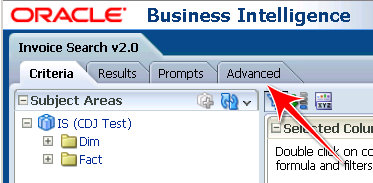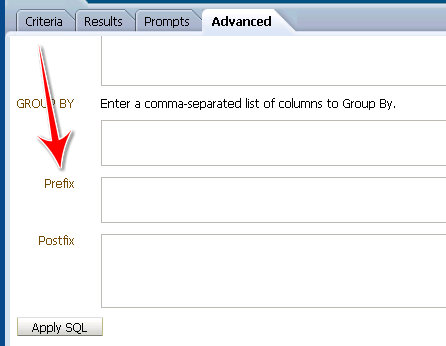## Thursday, August 11, 2011

### OBIEE: Stored Proc (Pipelined Function)

Last week I wrote up an issue with Session variables, you can read it here.

That was part of my project to use an Oracle pipelined function as a table source.

Initially, I was only accepting 4 parameters, but I had never gotten it to work perfectly.

Now I have 14 to work with and a deadline fast approaching.

Here's the function call:
```foo
( p_01 IN VARCHAR2,
p_02 IN VARCHAR2 DEFAULT NULL,
p_03 IN VARCHAR2 DEFAULT NULL,
p_04 IN VARCHAR2 DEFAULT NULL,
p_05 IN VARCHAR2 DEFAULT NULL,
p_06 IN VARCHAR2 DEFAULT NULL,
p_07 IN VARCHAR2 DEFAULT NULL,
p_08 IN VARCHAR2 DEFAULT NULL,
p_09 IN VARCHAR2 DEFAULT NULL,
p_10 IN VARCHAR2 DEFAULT NULL,
p_11 IN VARCHAR2 DEFAULT NULL,
p_12 IN VARCHAR2 DEFAULT NULL,
p_13 IN DATE DEFAULT NULL,
p_14 IN NUMBER DEFAULT NULL )
RETURN my_object_table PIPELINED;```
Here's the table source:
```SELECT *
FROM TABLE( FOO( 'VALUEOF(NQ_SESSION.S_P_01)', 'VALUEOF(NQ_SESSION.S_P_02)',
'VALUEOF(NQ_SESSION.S_P_03)', 'VALUEOF(NQ_SESSION.S_P_04)',
'VALUEOF(NQ_SESSION.S_P_05)', 'VALUEOF(NQ_SESSION.S_P_06)',
'VALUEOF(NQ_SESSION.S_P_07)', 'VALUEOF(NQ_SESSION.S_P_08)',
'VALUEOF(NQ_SESSION.S_P_09)', 'VALUEOF(NQ_SESSION.S_P_10)',
'VALUEOF(NQ_SESSION.S_P_11)', 'VALUEOF(NQ_SESSION.S_P_12)',
'VALUEOF(NQ_SESSION.S_P_13)', 'VALUEOF(NQ_SESSION.S_P_14)' )```
Note that everything is enclosed in single quotes.

On the report side, I navigate to the Advanced tabIn the Prefix boxI add the following (you'll have to assume that I have created my prompts and presentation variables already, I'm too lazy to go in and create them again).
```SET VARIABLE S_P_01='@{P_S_P_01}',S_P_02='@{P_S_P_02}',S_P_03='@{P_S_P_03}',
S_P_04='@{P_S_P_04}',S_P_05='@{P_S_P_05}',S_P_06='@{P_S_P_06}',S_P_07='@{P_S_P_07}',
S_P_08='@{P_S_P_08}',S_P_09='@{P_S_P_09}',S_P_10='@{P_S_P_10}',S_P_11='@{P_S_P_11}',
S_P_12='@{P_S_P_12}',S_P_13='@{P_S_P_13}',S_P_14='@{P_S_P_14}';```
(I put hard returns in there for display purposes, remove those).

All is well.

Or not.

I enter in a value in Prompt 1, P_S_P_01, and nothing for the rest. Take a look at the physical SQL:
```SELECT *
FROM TABLE( FOO( 'some value', '@{P_S_P_02}',
'@{P_S_P_03}', '@{P_S_P_04}',
'@{P_S_P_05}', '@{P_S_P_06}',
'@{P_S_P_07}', '@{P_S_P_08}',
'@{P_S_P_09}', '@{P_S_P_10}',
'@{P_S_P_11}', '@{P_S_P_12}',
'@{P_S_P_13}', '@{P_S_P_14}' )```
Yeah, that's not going to work. Off to Gerard Nico's excellent wiki. There I see you can have a default value for the presentation variable.
`@{variables.<variableName>}{<default>}[format]`
Excellent. At the end of each session variable assignment, I tack on {NULL}. That didn't work either. That just put NULL in single quotes, like this: 'NULL'. Barnacles.

I then asked for help. Frank suggested wrapping each call to the session variable in the SQL statement in a CASE statement (I swear I had tried that...), so I did...and it worked. Here's my final SQL (Table Source):
```SELECT *
FROM TABLE( FOO( 'VALUEOF(NQ_SESSION.S_P_01)',
( CASE WHEN 'VALUEOF(NQ_SESSION.S_P_01)' = 'NULL' THEN NULL ELSE 'VALUEOF(NQ_SESSION.S_P_01)' END ),
( CASE WHEN 'VALUEOF(NQ_SESSION.S_P_02)' = 'NULL' THEN NULL ELSE 'VALUEOF(NQ_SESSION.S_P_02)' END ),
( CASE WHEN 'VALUEOF(NQ_SESSION.S_P_03)' = 'NULL' THEN NULL ELSE 'VALUEOF(NQ_SESSION.S_P_03)' END ),
( CASE WHEN 'VALUEOF(NQ_SESSION.S_P_04)' = 'NULL' THEN NULL ELSE 'VALUEOF(NQ_SESSION.S_P_04)' END ),
( CASE WHEN 'VALUEOF(NQ_SESSION.S_P_05)' = 'NULL' THEN NULL ELSE 'VALUEOF(NQ_SESSION.S_P_05)' END ),
( CASE WHEN 'VALUEOF(NQ_SESSION.S_P_06)' = 'NULL' THEN NULL ELSE 'VALUEOF(NQ_SESSION.S_P_06)' END ),
( CASE WHEN 'VALUEOF(NQ_SESSION.S_P_07)' = 'NULL' THEN NULL ELSE 'VALUEOF(NQ_SESSION.S_P_07)' END ),
( CASE WHEN 'VALUEOF(NQ_SESSION.S_P_08)' = 'NULL' THEN NULL ELSE 'VALUEOF(NQ_SESSION.S_P_08)' END ),
( CASE WHEN 'VALUEOF(NQ_SESSION.S_P_09)' = 'NULL' THEN NULL ELSE 'VALUEOF(NQ_SESSION.S_P_09)' END ),
( CASE WHEN 'VALUEOF(NQ_SESSION.S_P_10)' = 'NULL' THEN NULL ELSE 'VALUEOF(NQ_SESSION.S_P_10)' END ),
( CASE WHEN 'VALUEOF(NQ_SESSION.S_P_11)' = 'NULL' THEN NULL ELSE 'VALUEOF(NQ_SESSION.S_P_11)' END ),
( CASE WHEN 'VALUEOF(NQ_SESSION.S_P_12)' = 'NULL' THEN NULL ELSE 'VALUEOF(NQ_SESSION.S_P_12)' END ),
( CASE WHEN 'VALUEOF(NQ_SESSION.S_P_13)' = 'NULL' THEN NULL ELSE 'VALUEOF(NQ_SESSION.S_P_13)' END ),
( CASE WHEN 'VALUEOF(NQ_SESSION.S_P_14)' = 'NULL' THEN NULL ELSE 'VALUEOF(NQ_SESSION.S_P_14)' END ) ) )```
Absolutely hideous! And annoying. But it works.

Hopefully someone (hint hint) will chime in with a better method.